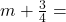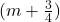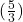## It takes Ricky, traveling at 24 mph, 45 minutes longer to go a certain distance than it takes Maria traveling at 51 mph, Find the dis

Question

It takes Ricky, traveling at 24 mph, 45 minutes longer to go a certain distance than it takes Maria traveling at 51 mph, Find the distance traveled.

in progress 0
7 months 2021-08-01T16:49:14+00:00 1 Answers 8 views 0

85 mi

Step-by-step explanation:

Let d = the distance in miles traveled

Let M = the time in hours for Maria to travel d milestime in hours for Ricky to travel d miles

(Note thathrs = 45 min)

———————-

Maria’s equation:

d = 51m

Ricky’s equation:

d = 24 ·———————-

Substitution:

51m = 24 ·51m = 24m + 45

6m = 10

m =———————-

d = 51m

d = 51 ·d = 85

———————-

The distance traveled is 85 mi